# AQA A Level Maths: Pure复习笔记6.2.1 Laws of Logarithms

### Laws of Logarithms

#### What are the laws of logarithms?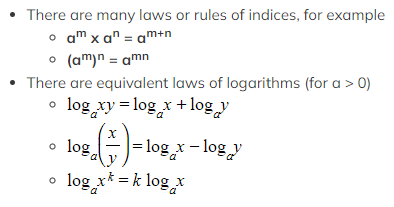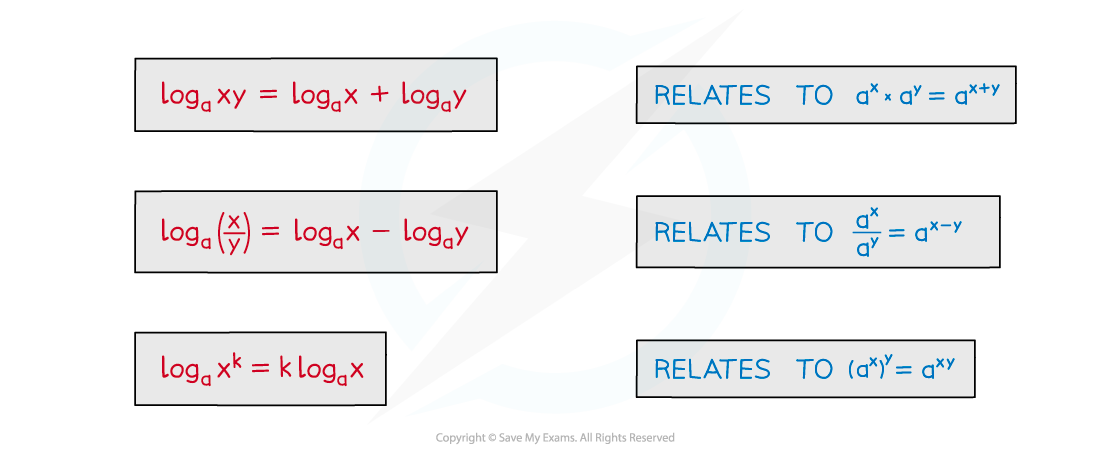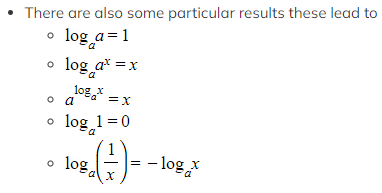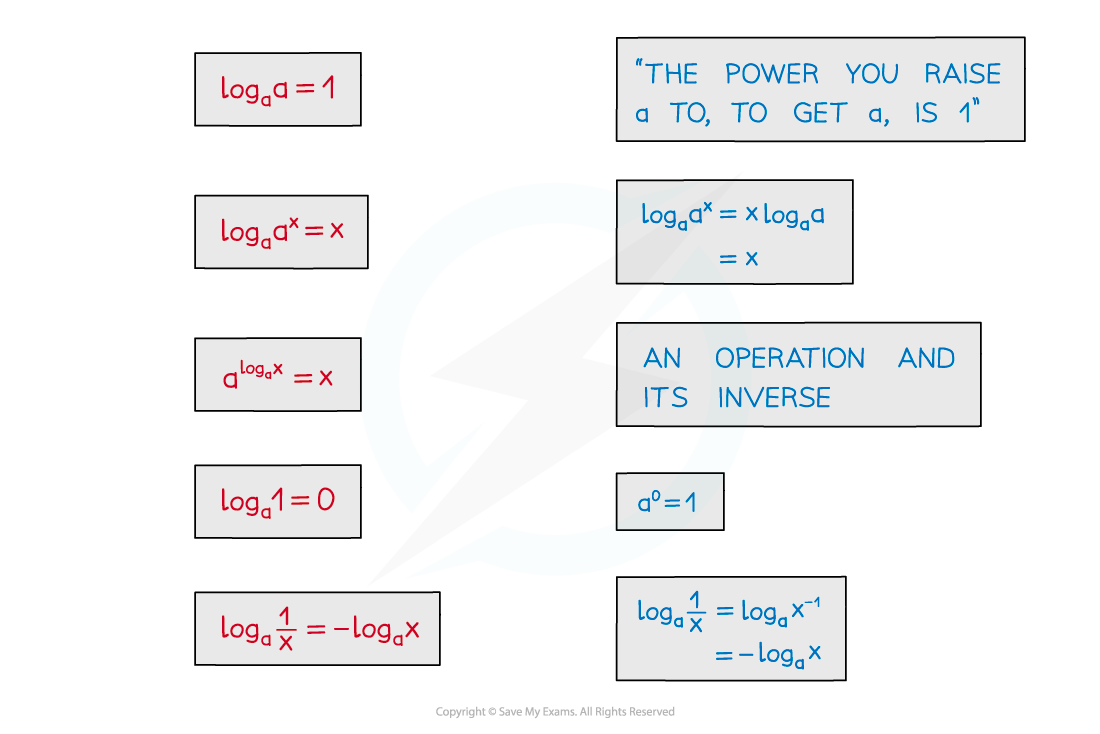• Two of these were seen in the notes Logarithmic Functions
• Beware …
• … log (x + y) ≠ log x + log y
• Results apply to ln too

####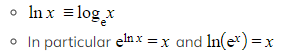How do I use the laws of logarithms?

• Laws of logarithms can be used to …
• … simplify expressions
• … solve logarithmic equations
• … solve exponential equations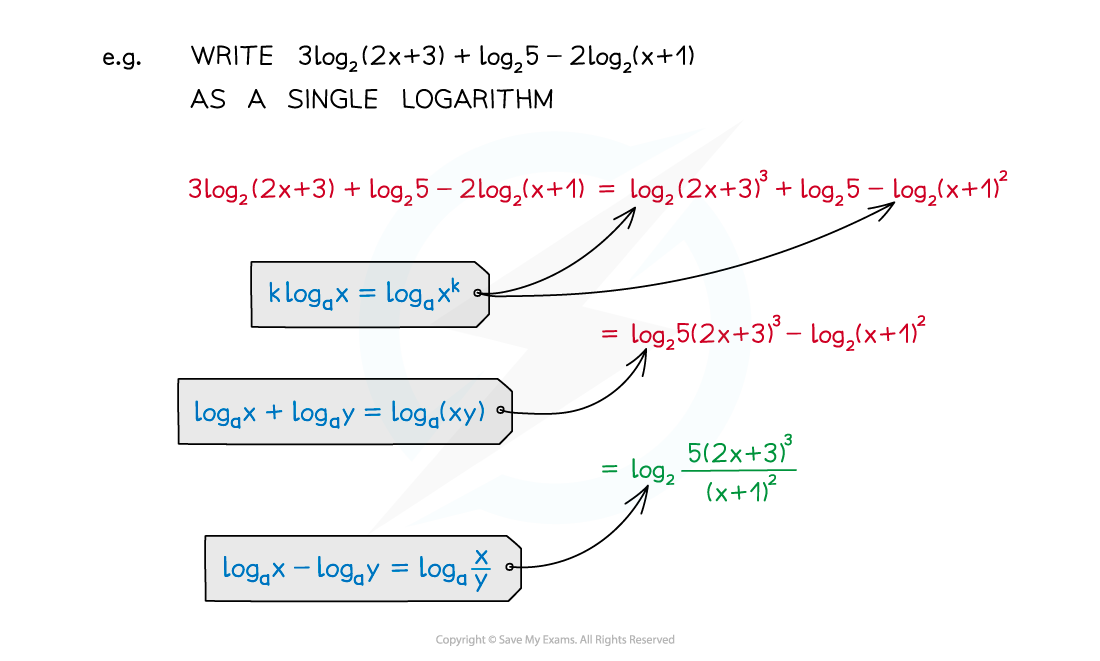#### Exam Tip

• Remember to check whether your solutions are valid
• log (x+k) is only defined if x > -k
• You will lose marks if you forget to reject invalid solutions

#### Worked Example